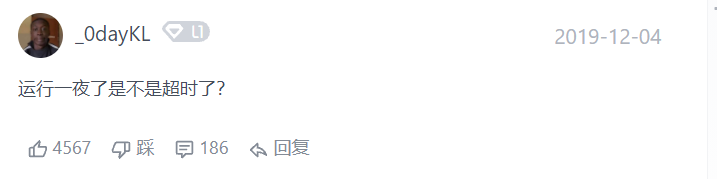刷题：LeetCode企业某节跳动排序前200道题--每周更新1、两数之和

代码
class Solution {
public int[] twoSum(int[] nums, int target) {

Map<Integer, Integer> map = new HashMap();

int[] res = new int;

for(int i = 0; i < nums.length; i++) {
if(map.containsKey(nums[i])) {
res = i;
res = map.get(nums[i]);
return res;
} else {
map.put(target - nums[i], i);
}
}
return res;
}
}
趣评---该题点赞最多的前两条评论：2、两数相加

代码：
class Solution {
public ListNode addTwoNumbers(ListNode l1, ListNode l2) {

int carry = 0;

while(l1 != null || l2 != null || carry != 0) {

if(l1 != null) {
carry = carry + l1.val;
l1 = l1.next;
}
if(l2 != null) {
carry = carry + l2.val;
l2 = l2.next;
}

pre.next = new ListNode(carry % 10);
pre = pre.next;
carry =  carry / 10;
}